﻿ 基于含权Newman算法的交通控制子区划分

# 基于含权Newman算法的交通控制子区划分Traffic Control Subarea Partition Based on Weighted Newman Algorithms

1. 引言

2. Newman快速划分算法及其改进

$Q=\underset{ij}{\sum }\left[\frac{{A}_{ij}}{2m}-\frac{{k}_{i}*{k}_{j}}{\left(2m\right)\left(2m\right)}\right]{\delta }_{\left({C}_{i},{C}_{i}\right)}$ (1)

$Q=\underset{i}{\sum }\left({e}_{ii}-{a}_{i}{}^{2}\right)=\text{Tre}-‖{e}^{2}‖$ (2)

${e}^{2}=\underset{i}{\sum }{a}_{i}^{2}=\underset{i}{\sum }{\sum }_{j,k}{e}_{ij}{e}_{ik}$ (3)

2.1. 基于模块度的Newman快速划分算法

2.2. 算法步骤

Newman快速算法执行过程如下：

1) 首先把含有 $n$ 个的节点复杂网络视为 $n$ 个社团，那么，初始时有：

 (4)

${a}_{i}=\frac{{k}_{i}}{2m}$ (5)

2) 根据贪婪算法的原理，沿着使 $Q$ 增加或者减小速度最小的方向，依次合并两个有边连接的社团，并计算合并后的 $Q$ 值增量：

$\Delta Q={e}_{ij}+{e}_{ji}-2{a}_{i}{a}_{j}=2\left({e}_{ij}-{a}_{i}{a}_{j}\right)$ (6)

3) 重复上一步，直到每个节点都合并成为一个社团为止。

2.3. 算法改进

${D}_{ij}=1-\frac{|{d}_{i}-{d}_{j}|}{{\sum }^{\text{​}}{d}_{i}}$ (7)

${e}_{ij}=\left\{\begin{array}{l}\frac{{D}_{ij}}{{\sum }_{i,j}{D}_{ij}};节点i与节点j之间有边相连\\ 0;其他\end{array}$ (8)

${a}_{i}$ 可以表示为：

${a}_{i}=\frac{{\sum }_{j}{e}_{ij}}{{\sum }_{i,j}{D}_{ij}}$ (9)

3. 实例分析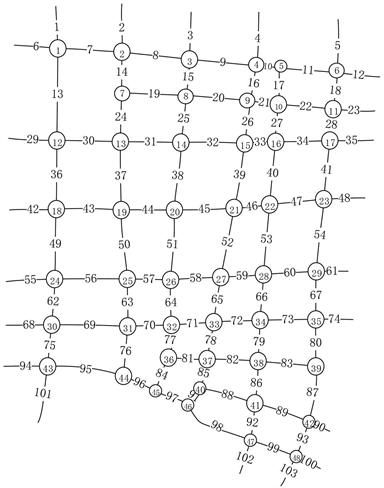Figure 1. Changde downtown urban road network map

3.1. 子区划分结果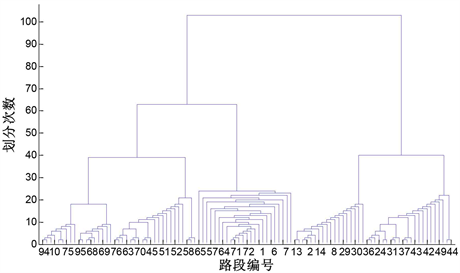Figure 2. Controls sub-area partitioning tree diagram based on Traditional Newman algorithm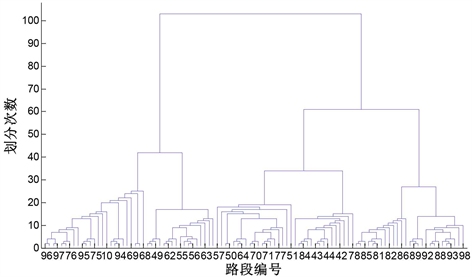Figure 3. Controls sub-area partitioning tree diagram based on Improved Newman algorithm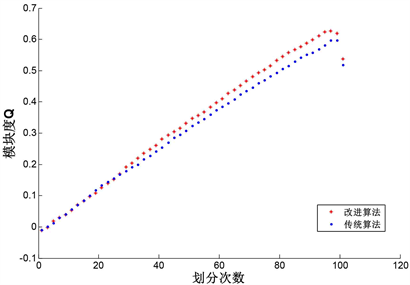Figure 4. Modular values of subdivision results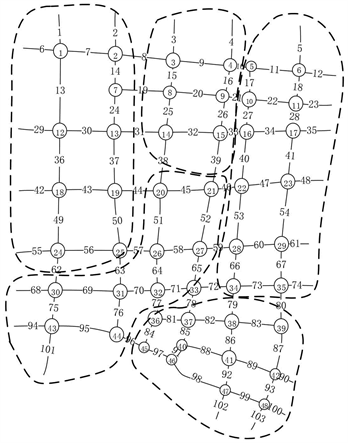Figure 5. Control sub-area division result based on Traditional Newman algorithm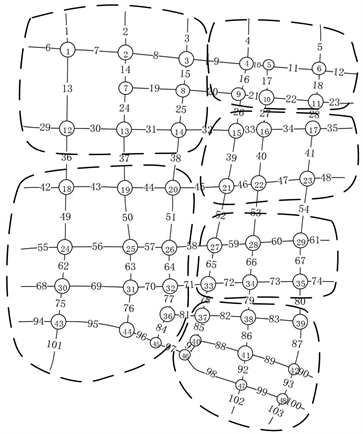Figure 6. Control sub-area division result based on Improved Newman algorithm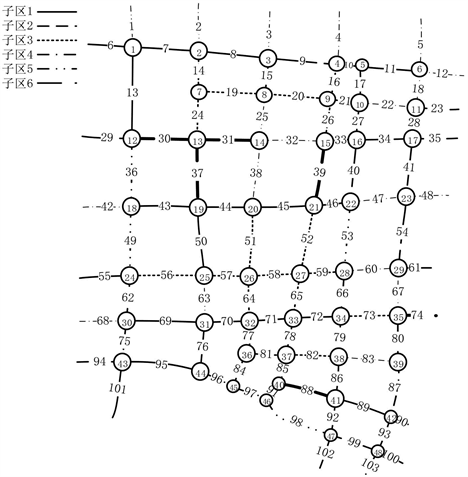Figure 7. First sub-division based on K-means clustering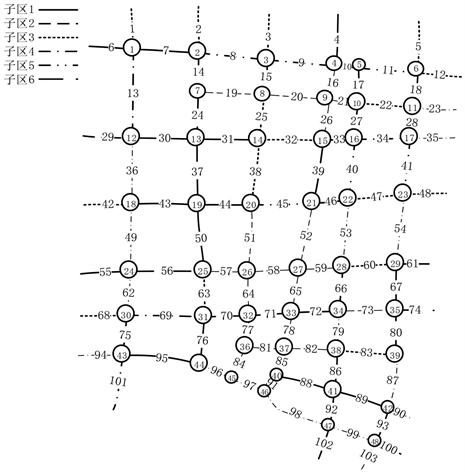Figure 8. Second sub-division based on K-means clustering

3.2. 划分结果指标分析

$N{S}_{k}\left(A,B\right)=\frac{{\sum }_{i\in A}{\sum }_{j\in B}{\left({d}_{i}-{d}_{j}\right)}^{2}}{{N}_{A}{N}_{B}}$ (10)

${d}_{i}$ 表示路段 $i$ 的密度， ${N}_{A},{N}_{B}$ 分别为子区域A和子区域B路段的数目。由于 $N{S}_{k}\left(A,B\right)$ 仅表征了子区域A和子区域B之间的平均密度距离，并不能体现子区之间的空间关系。为了补充这一点，进而采用公式(11)对其进行评价：

$N{S}_{k}\left(A\right)=\frac{N{S}_{k}\left(A,A\right)}{N{S}_{k}\left(A,B\right)}$ (11)

$N{S}_{k}\left(A,B\right)=\mathrm{min}\left\{N{S}_{k}\left(A,K\right)|K\in Neighbor\left(A\right)\right\}$ (12)

$AN{S}_{k}=\frac{{\sum }_{A\in C}N{S}_{k}\left(A\right)}{k}$ (13)

$NS$ 评价标准也可以以路段拟交通流密度的方差和均值来表达：

$N{S}_{k}\left(A,B\right)=Var\left(A\right)+Var\left(B\right)+{\left({u}_{A}-{u}_{B}\right)}^{2}$ (14)

$N{S}_{k}\left(A\right)=\frac{N{S}_{k}\left(A,A\right)}{N{S}_{k}\left(A,B\right)}=\frac{2Var\left(A\right)}{Var\left(A\right)+Var\left(B\right)+{\left({u}_{A}-{u}_{B}\right)}^{2}}$ (15)Table 1. Evaluation index of various zoning methods

4. 小结

 Walinchus, R.J. (1971) Real-Time Network Decomposition and Sub Network Interfacing. Highway Research Record, 7, 20-28.

 Ji, Y. and Geroliminis, N. (2012) On the Spatial Partitioning of Urban Transportation Networks. Transportation Research Part B: Methodological, 16, 1639-1656.
https://doi.org/10.1016/j.trb.2012.08.005

 Ji, Y. and Geroliminis, N. (2011) Spatial and Temporal Analysis of Congestion in Urban Transportation Networks. Trans-portation Research Board Annual Meeting, 1791-1808.

 李刚奇, 赵娅丽. 基于宏观交通理论的交通控制子区划分方法[C]//第七届中国智能交通年会. 第七届中国智能交通年会优秀论文集——智能交通技术. 北京: 中国智能交通协会, 2012: 31-38.

 马莹莹, 杨晓光, 曾澄. 基于谱方法的城市交通信号控制网络小区划分方法[J]. 系统工程理论与实践, 2010, 30(12): 2290-2296.

 卢守峰, 陶黎明, 江勇东. 考虑连接性的路网划分算法[J]. 交通运输系统工程与信息, 2018, 18(5): 95-102.

 Dimitriou, L. and Nikolaou, P. (2017) Dynamic Partitioning of Urban Road Networks Haled on Their Topological Andoperational Characteristics. IEEE International Conference on MODELS and Technologies for Intelligent Transportation Systems, Naples, Italy, 26-28 June 2017, 457-462.

 王晓轩. 基于聚类的城市交通路网分区和交通状态判别[D]: [硕士学位论文]. 北京: 北京交通大学, 2017.

 Newman, M.E.J. and Girvan, M. (2004) Finding and Evaluating Community Structure in Networks. Physical Review E, 69, Article ID: 026113.
https://doi.org/10.1103/PhysRevE.69.026113

 Girvan, M. and Newman, M.E.J. (2002) Community Structure in Social and Biological Networks. Proceedings of the National Academy of Sciences of the United States of America, 99, 7821-7826.
https://doi.org/10.1073/pnas.122653799

 Ji, Y.X. and Geroliminis N. (2012) On the Spatial Partitioning of Urban Transportation Networks. Transportation Research Part B, 46, 1639-1656.
https://doi.org/10.1016/j.trb.2012.08.005

 Kernighan, B.W. and Lin, S. (1970) An Efficient Heuristic Procedure for Partitioning Graphs. The Bell System Technical Journal, 49, 291-307.
https://doi.org/10.1002/j.1538-7305.1970.tb01770.x

 Newman, M.E.J. (2004) Detecting Community Structure in Networks. European Physical Journal B, 38, 321-330.
https://doi.org/10.1140/epjb/e2004-00124-y

 刘澜, 卢维科, 胡国静, 等. 面向边界控制地路网小区划分[J]. 中国公路学报, 2018, 31(11): 187-196.

Top